The EOQ Model with Shortages[Page 742 ( continued )]

One of the assumptions of our basic EOQ model is that shortages and back ordering are not allowed. The third model variation that we will describe, the EOQ model with shortages, relaxes this assumption. However, it will be assumed that all demand not met because of inventory shortage can be back ordered and delivered to the customer later. Thus, all demand is eventually met. The EOQ model with shortages is illustrated in Figure 16.7.

(This item is displayed on page 743 in the print version)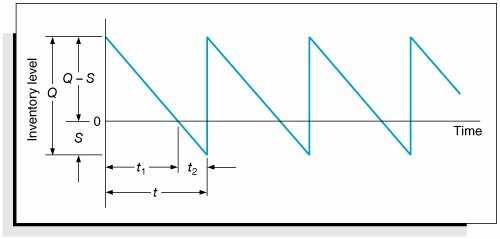The EOQ model with shortages relaxes the assumption that shortages cannot exist .

Because back-ordered demand, or shortages, S , are filled when inventory is replenished, the maximum inventory level does not reach Q , but instead a level equal to Q S . It can be seen from Figure 16.7 that the amount of inventory on hand ( Q S ) decreases as the amount of the shortage increases , and vice versa. Therefore, the cost associated with shortages, which we described earlier in this chapter as primarily the cost of lost sales and lost customer goodwill, has an inverse relationship to carrying costs. As the order size , Q , increases, the carrying cost increases and the shortage cost declines. This relationship between carrying and shortage cost as well as ordering cost is shown in Figure 16.8.

[Page 743]
Figure 16.8. Cost model with shortages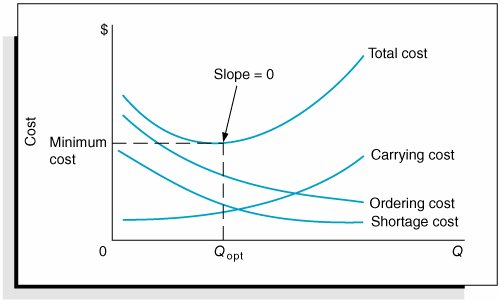We will forgo the lengthy derivation of the individual cost components of the EOQ model with shortages, which requires the application of plane geometry to the graph in Figure 16.8. The individual cost functions are provided as follows , where S equals the shortage level and C s equals the annual per-unit cost of shortages: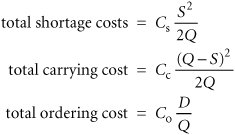Combining these individual cost components results in the total inventory cost formula: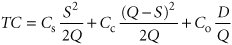[Page 744]

You will notice in Figure 16.8 that the three cost component curves do not intersect at a common point, as was the case in the basic EOQ model. As a result, the only way to determine the optimal order size and the optimal shortage level , S , is to differentiate the total cost function with respect to Q and S , set the two resulting equations equal to zero, and solve them simultaneously . Doing so results in the following formulas for the optimal order quantity and shortage level: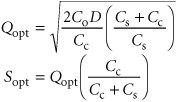For example, we will now assume that the I-75 Carpet Discount Store allows shortages and the shortage cost, C s , is \$2 per yard per year. All other costs and demand remain the same ( C c = \$0.75, C o = \$150, and D = 10,000 yd.). The optimal order size and shortage level and total minimum annual inventory cost are computed as follows: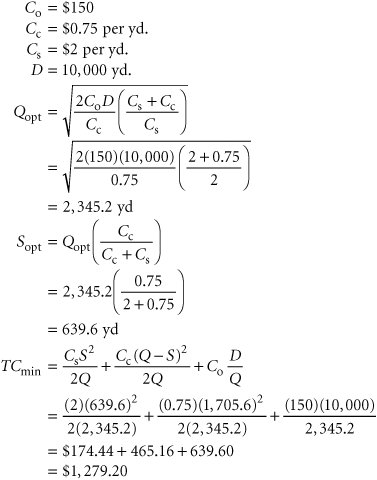Several additional parameters of the EOQ model with shortages can be computed for this example, as follows: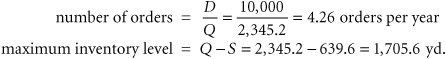[Page 745]

The time between orders, identified as t in Figure 16.7, is computed as follows: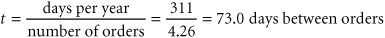The time during which inventory is on hand, t 1 in Figure 16.7, and the time during which there is a shortage, t 2 in Figure 16.7, during each order cycle can be computed using the following formulas: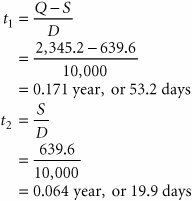Management Science Application: Determining Inventory Ordering Policy at Dell

Dell Inc., the world's largest computer-systems company, bypasses retailers and sells directly to customers via phone or the Internet. After an order is processed , it is sent to one of its assembly plants in Austin, Texas, where the product is built, tested , and packaged within 8 hours.

Dell carries very little components inventory itself. Technology changes occur so fast that holding inventory can be a huge liability; some components lose 0.5% to 2.0% of their value per week. In addition, many of Dell's suppliers are located in Southeast Asia, and their shipping times to Austin range from 7 days for air transport to 30 days for water and ground transport. To compensate for these factors, Dell's suppliers keep inventory in small warehouses called "revolvers" (for revolving inventory), which are a few miles from Dell's assembly plants. Dell keeps very little inventory at its own plants, so it withdraws inventory from the revolvers every few hours, while most Dell's suppliers deliver to their revolvers three times per week.

The cost of carrying inventory by Dell's suppliers is ultimately reflected in the final price of a computer. Thus, in order to maintain a competitive price advantage in the market, Dell strives to help its suppliers reduce inventory costs. Dell has a vendor-managed inventory (VMI) arrangement with its suppliers, which decide how much to order and when to send their orders to the revolvers. Dell's suppliers order in batches (to offset ordering costs), using a continuous ordering system with a batch order size, Q , and a reorder point, R , where R is the sum of the inventory on order and a safety stock. The order size estimate, based on long- term data and forecasts, is held constant. Dell sets target inventory levels for its supplierstypically 10 days of inventoryand keeps track of how much suppliers deviate from these targets and reports this information back to suppliers so that they can make adjustments accordingly .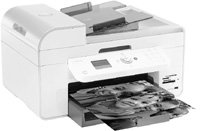Source: R. Kapuscinski, R. Zhang, P. Carbonneau, R. Moore, and B. Reeves, "Inventory Decisions in Dell's Supply Chain," Interfaces 34, no. 3 (MayJune 2004): 191205.Introduction to Management Science (10th Edition)
ISBN: 0136064361
EAN: 2147483647
Year: 2006
Pages: 358

Similar book on Amazon